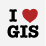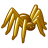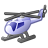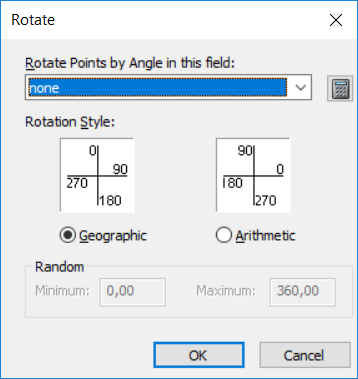# How can I convert aritmetic degree to geographic?

12225
9
10-07-2014 06:43 AMNew Contributor II

The difference of the two has been explained in another post:

"A note for those not familiar with rotation options.  Geographic rotation establishes due north as angle 0 and degrees above 0 cause a clockwise rotation so that due east is 90, due south is 180 and due west is 270.  It's rules are designed to operate like a clock.  An Arithmetic rotation follows Cartesian graphing rules and is what mathematicians use.  It establishes the X axis in the positive direction (X = +, Y = 0) or due east in compass directions as 0 and degrees above 0 cause a counterclockwise rotation.  In an arithmetic system due north is 90 degrees (X = 0, Y = +), due west is 180 degrees (X = -, Y = 0), and due south is 270 degrees (X = 0, Y = -)."

The concept is clear to me... but how can I caculate one from the other? As I have aritmetic direction in my data, but I need them in geographic degree.

Thank you! Frauke

Tags (3)
9 RepliesOccasional Contributor III

Have a look here, quite old:New Contributor II

Dear Johannes,

I found the old post too, but the script logically does not work for me. Maybe I have some missunderstanding.

But if I try to apply this script to my arithmetic value of f.e. 270°, which is pointing to the west:

270 + 270 = 540

this is > 360: 540 - 360 = 180°

... and it should be 90° in geometric direction?!? This script is just 'shifting' the values, but not taking into account the 'counter clock rotation' of the arimetic direction. As I am not that much into VBA I might miss something here.

Thank you!byMVP Legendary Contributor

Fire up python and go all command line

>>> import math

>>> pnt_1 = [1.0,-1.0]

>>> pnt_0 = [0.0,0.0]

>>> radian = math.atan((pnt_1 - pnt_0)/(pnt_1 - pnt_0))

-0.7853981633974483

>>> if degrees < 0.0:

...  degrees += 360

...

>>> degrees

315.0

>>>

If you want to determine the azimuth of a polyline, then you can use this field calculator function

```''' input shape field: returns angle between 0 and <360 based upon the first and last point
polyline_azimuth(!Shape!)    #enter into the expression box'''
import math
def polyline_azimuth(shape):
radian = math.atan((shape.lastPoint.X - shape.firstPoint.X)/(shape.lastPoint.Y - shape.firstPoint.Y))
if degrees <0:
return degrees + 360
else:
return degrees
```New Contributor

Well, it seems to be that this post is almost 2 years old. Nevertheless there is an easier way to calculate from arithmetic into geographic degrees.

This is what I got:

(Calculation in ArcMap - Phyton)

def CalcGeograpicAngle(arith):

if (arith >90 and arith <360):

return (360-arith+90)

elif (arith>=0 and arith <= 90):

return (arith*(-1)+90)

It worked for me and the vice versa should be also easy like that.New Contributor III

I think a cleaner solution would be a single expression.

def CalcGeographicAngle(arith):

return (360 - arith + 90) % 360byMVP Legendary Contributor

or...  (450-arith) % 360byMVP Regular Contributor

Yeah, this modulo operation (%) is cool! See: https://en.wikipedia.org/wiki/Modulo_operation

And, by the way, the formula works both ways, so both from Arithmetic to Geographic and from Geographic to Arithmetic, just like a switch:

``````# e.g. Geographic North (12 o'clock) = 0

(450 - 0) % 360‍‍‍ # returns 90 (Arithmetic)

# e.g. Arithmetic South (6 o'clock) = 270

(450 - 270) % 360 # returns 180 (Geographic)‍‍‍‍‍‍‍``````New Contributor III

Derek Nalder's way:

``````def CalcGeographicAngle(arith):
return (360 - arith + 90) % 360‍‍``````

is actually the more "pythonic" way of doing this. It is better to be explicit then implicit, and I think it is much easier to understand what is happening with his version.byMVP Legendary Contributor

I suppose... but if you rotate a circle by 90 degrees, the origin vector has moved through 450 degrees.  Think of the arm of a clock, it travels 450 degrees in an hour and 15 minutes